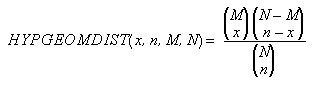﻿ HYPGEOMDIST | Spread Formula Reference
Formula Functions / Functions H to L / HYPGEOMDIST
In This Topic
HYPGEOMDIST
In This Topic

This function returns the hypergeometric distribution.

#### Syntax

HYPGEOMDIST(x,n,M,N)

#### Arguments

The arguments are as follows, and are truncated if not integers:

Argument Description
x An integer representing the number of successes in the sample
n An integer representing the size of the sample
M An integer representing the number of successes in the population
N An integer representing the size of the population

#### Remarks

The equation for this function is:#### Data Types

Accepts numeric data for all arguments. Returns numeric data.

#### Examples

HYPGEOMDIST(A22,B23,62,1000)

HYPGEOMDIST(R22C11,R22C12,R34C14,R35C15)

HYPGEOMDIST(2,37,6,100) gives the result 0.3327981975

#### Version Available

This function is available in product version 1.0 or later.